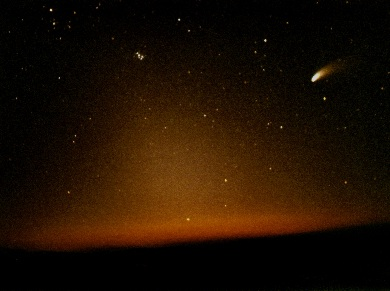A few definitions...Glossary Astronomical Unit (AU): Unit of distance. Defined as the distance between Earth and the Sun, or 1.496 x 10^8 kilometer. Circumstellar: Adjective for something that "surrounds a star". If something surrounds two stars, circumstellar becomes "circumbinary". Earth Mass (M_earth): 5.974 x 10^24 kilograms Extrasolar or Exosolar: Planets or objects orbiting stars other than the Sun. For example, an extrasolar planet is a planet around another star. Jupiter Mass (M_jup): 1.899 x 10^27 kilograms Lunar Mass (M_lunar): 7.349 x 10^22 kilograms Meter (m): The base unit of length in the International System of Units. Equal to about 39.37 inches. Micron: Unit of distance, one million times smaller than a meter. Millimeter (mm): Unit of distance, one thousand times smaller than a meter. Parsec (pc): Unit of distance. 3.09 x 10^13 km or 206265 AU or 3.3 light year Solar: Pertaining to the Sun. Solar Luminosity (L_sun): 3.90 x 10^26 Watts Solar Mass (M_sun): 1.99 x 10^30 kilograms Solar Radius (R_sun): 6.96 x 10^8 meter